Test: Heron`s Formula- Case Based Type Questions

# Test: Heron`s Formula- Case Based Type Questions - Class 9

Test Description

## 10 Questions MCQ Test Additional Practice for Class 9 - Test: Heron`s Formula- Case Based Type Questions

Test: Heron`s Formula- Case Based Type Questions for Class 9 2023 is part of Additional Practice for Class 9 preparation. The Test: Heron`s Formula- Case Based Type Questions questions and answers have been prepared according to the Class 9 exam syllabus.The Test: Heron`s Formula- Case Based Type Questions MCQs are made for Class 9 2023 Exam. Find important definitions, questions, notes, meanings, examples, exercises, MCQs and online tests for Test: Heron`s Formula- Case Based Type Questions below.
Solutions of Test: Heron`s Formula- Case Based Type Questions questions in English are available as part of our Additional Practice for Class 9 for Class 9 & Test: Heron`s Formula- Case Based Type Questions solutions in Hindi for Additional Practice for Class 9 course. Download more important topics, notes, lectures and mock test series for Class 9 Exam by signing up for free. Attempt Test: Heron`s Formula- Case Based Type Questions | 10 questions in 20 minutes | Mock test for Class 9 preparation | Free important questions MCQ to study Additional Practice for Class 9 for Class 9 Exam | Download free PDF with solutions
 1 Crore+ students have signed up on EduRev. Have you?
Test: Heron`s Formula- Case Based Type Questions - Question 1

### Direction: Isosceles triangles were used to construct a bridge in which the base (unequal side) of an isosceles triangle is 4 cm and its perimeter is 20 cm.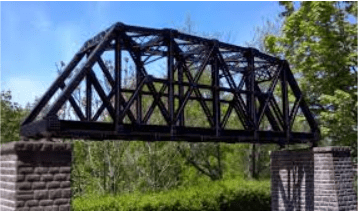Q. What is the length of equal sides?

Detailed Solution for Test: Heron`s Formula- Case Based Type Questions - Question 1
Let x cm be the length of equal sides of the isosceles triangle.

So, x + x + 4 = 20

2x + 4 = 20

2x = 20 – 4

2x = 16

x = 16/2 = 8 cm.

Test: Heron`s Formula- Case Based Type Questions - Question 2

### Direction: Isosceles triangles were used to construct a bridge in which the base (unequal side) of an isosceles triangle is 4 cm and its perimeter is 20 cm.Q. If the sides of a triangle are in the ratio 3 : 5 : 7 and its perimeter is 300 m. Find its area.

Detailed Solution for Test: Heron`s Formula- Case Based Type Questions - Question 2

Let the sides of a triangle are a = 3x, b = 5x, c = 7x

Then a + b + c = 300

3x + 5x + 7x = 300

15x = 300

x = 20

So, a = 60, b = 100, c = 140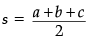= 300/2 = 150

Area of triangle =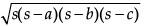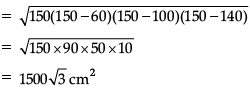Test: Heron`s Formula- Case Based Type Questions - Question 3

### Direction: Isosceles triangles were used to construct a bridge in which the base (unequal side) of an isosceles triangle is 4 cm and its perimeter is 20 cm.Q. What is the Heron's formula for the area of?

Detailed Solution for Test: Heron`s Formula- Case Based Type Questions - Question 3
Area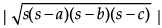{Area} = area

s = semi-perimeter

a = length of side a

b = length of side b

c = length of side c

Test: Heron`s Formula- Case Based Type Questions - Question 4

Direction: Isosceles triangles were used to construct a bridge in which the base (unequal side) of an isosceles triangle is 4 cm and its perimeter is 20 cm.Q. What is the semi perimeter of the highlighted triangle?

Detailed Solution for Test: Heron`s Formula- Case Based Type Questions - Question 4
Required semi perimeter = Perimeter/2 = 20/2 = 10 m.
Test: Heron`s Formula- Case Based Type Questions - Question 5

Direction: Isosceles triangles were used to construct a bridge in which the base (unequal side) of an isosceles triangle is 4 cm and its perimeter is 20 cm.Q. What is the area of highlighted triangle ?

Detailed Solution for Test: Heron`s Formula- Case Based Type Questions - Question 5
Since, semi perimeter, s = 10 cm

Thus, area of the triangle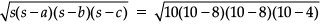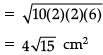Test: Heron`s Formula- Case Based Type Questions - Question 6

Direction: Shakshi prepared a Rangoli in triangular shape on Diwali. She makes a small triangle under a big triangle as shown in figure.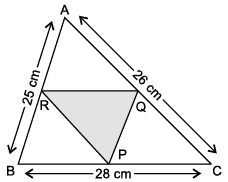Sides of big triangle are 25 cm, 26 cm and 28 cm. Also, ΔPQR is formed by joining mid points of sides of ΔABC.

Use the above data to help her in resolving below doubts.

Q. What is the semi-perimeter of ΔABC?

Detailed Solution for Test: Heron`s Formula- Case Based Type Questions - Question 6
P = sum of all 3 sides

= (25 + 26 + 28) cm = 79 cm

Test: Heron`s Formula- Case Based Type Questions - Question 7

Direction: Shakshi prepared a Rangoli in triangular shape on Diwali. She makes a small triangle under a big triangle as shown in figure.Sides of big triangle are 25 cm, 26 cm and 28 cm. Also, ΔPQR is formed by joining mid points of sides of ΔABC.

Use the above data to help her in resolving below doubts.

Q. Area of ΔPQR =

Detailed Solution for Test: Heron`s Formula- Case Based Type Questions - Question 7
Area of ΔPQR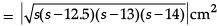Test: Heron`s Formula- Case Based Type Questions - Question 8

Direction: Shakshi prepared a Rangoli in triangular shape on Diwali. She makes a small triangle under a big triangle as shown in figure.Sides of big triangle are 25 cm, 26 cm and 28 cm. Also, ΔPQR is formed by joining mid points of sides of ΔABC.

Use the above data to help her in resolving below doubts.

Q. ½ of AB =

Detailed Solution for Test: Heron`s Formula- Case Based Type Questions - Question 8
½ of AB = QP
Test: Heron`s Formula- Case Based Type Questions - Question 9

Direction: Shakshi prepared a Rangoli in triangular shape on Diwali. She makes a small triangle under a big triangle as shown in figure.Sides of big triangle are 25 cm, 26 cm and 28 cm. Also, ΔPQR is formed by joining mid points of sides of ΔABC.

Use the above data to help her in resolving below doubts.

Q. What is the length of RQ?

Detailed Solution for Test: Heron`s Formula- Case Based Type Questions - Question 9
Length of RQ = ½ of BC

= ½ x 28

= 14 cm

Test: Heron`s Formula- Case Based Type Questions - Question 10

Direction: Shakshi prepared a Rangoli in triangular shape on Diwali. She makes a small triangle under a big triangle as shown in figure.Sides of big triangle are 25 cm, 26 cm and 28 cm. Also, ΔPQR is formed by joining mid points of sides of ΔABC.

Use the above data to help her in resolving below doubts.

Q. If colourful rope is to be placed along the sides of small ΔPQR. What is the length of the rope?

Detailed Solution for Test: Heron`s Formula- Case Based Type Questions - Question 10
Length of rope = Perimeter of ΔPQR

= (12.5 + 13 + 14) cm

= 39.5 cm

## Additional Practice for Class 9

266 docs|65 tests
Information about Test: Heron`s Formula- Case Based Type Questions Page
In this test you can find the Exam questions for Test: Heron`s Formula- Case Based Type Questions solved & explained in the simplest way possible. Besides giving Questions and answers for Test: Heron`s Formula- Case Based Type Questions, EduRev gives you an ample number of Online tests for practice

## Additional Practice for Class 9

266 docs|65 tests